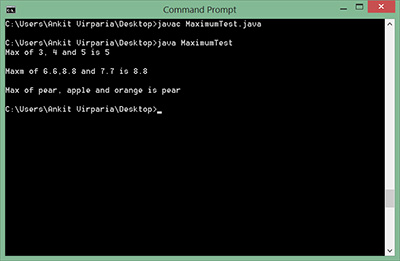In a simple terms, Generic provides you a freedom to pass the “Type” parameter when we create the object of such class.

Now, Assume that: A Generic class is written a developer and some other developers are gonna use the same(Your .class file). And you also want to restrict the other developers to pass some special kinds of types that are allowed as a type parameter. I mean – You don’t want to accept anything. Your class should accept only certain predefined things. How can we do that? The answer to this is “Bounded Type Generic Parameters”.

To declare a bounded type parameter, write the type parameter’s name, followed by the extends keyword, followed by its upper(parent) bound(class).

### Example:

We need a generic method of a generic class which only accepts three Comparable objects and return the largest object.

```public class MaximumTest
{
// determines the largest of three Comparable objects
public static <T extends Comparable<T>> T maximum(T x, T y, T z)
{
T max = x; // assume x is initially the largest
if ( y.compareTo( max ) > 0 ){
max = y; // y is the largest so far
}
if ( z.compareTo( max ) > 0 ){
max = z; // z is the largest now
}
return max; // returns the largest object
}
public static void main( String args[] )
{
System.out.printf( "Max of %d, %d and %d is %d\n\n", 3, 4, 5, maximum( 3, 4, 5 ) );

System.out.printf( "Maxm of %.1f,%.1f and %.1f is %.1f\n\n", 6.6, 8.8, 7.7, maximum( 6.6, 8.8, 7.7 ) );

System.out.printf( "Max of %s, %s and %s is %s\n","pear", "apple", "orange", maximum( "pear", "apple", "orange" ) );
}
}
```

Output:Note: Integer, Float, Boolean, String all the wrapper classes are implementing Comparable interface. That’s why maximum() method is accepting our arguments.

Let’s test it by passing StringBuffer objects which are not comparable because StringBuffer do not implements Comparable interface.

```public class MaximumTest
{
// determines the largest of three Comparable objects
public static <T extends Comparable<T>> T maximum(T x, T y, T z)
{
T max = x; // assume x is initially the largest
if ( y.compareTo( max ) > 0 ){
max = y; // y is the largest so far
}
if ( z.compareTo( max ) > 0 ){
max = z; // z is the largest now
}
return max; // returns the largest object
}
public static void main( String args[] )
{
StringBuffer s1 = new StringBuffer("Test");
StringBuffer s2 = new StringBuffer("Test2");
StringBuffer s3 = new StringBuffer("Test3");
maximum( s1, s2, s3 );
}
}
```